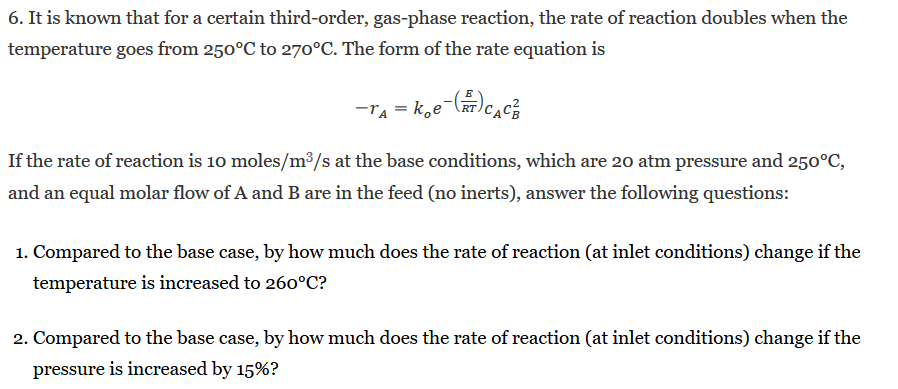# Question 6. It is known that for a certain third-order, gas-phase reaction, the rate of reaction doubles when the temperature goes from 250°C to 270°C. The form of the rate equation is If the rate of reaction is 10 moles/m3/s at the base conditions, which are 20 atm pressure and 250°C, and an equal molar flow of A and B are in the feed (no inerts), answer the 6. It is known that for a certain third-order, gas-phase reaction, the rate of reaction doubles when the temperature goes from 250°C to 270°C. The form of the rate equation is If the rate of reaction is 10 moles/m3/s at the base conditions, which are 20 atm pressure and 250°C, and an equal molar flow of A and B are in the feed (no inerts), answer the following questions 1. Compared to the base case, by how much does the rate of reaction (at inlet conditions) change if the temperature is increased to 260°C? 2. Compared to the base case, by how much does the rate of reaction (at inlet conditions) change if the pressure is increased by 15%?UFBK2V The Asker · Chemical EngineeringTranscribed Image Text: 6. It is known that for a certain third-order, gas-phase reaction, the rate of reaction doubles when the temperature goes from 250°C to 270°C. The form of the rate equation is If the rate of reaction is 10 moles/m3/s at the base conditions, which are 20 atm pressure and 250°C, and an equal molar flow of A and B are in the feed (no inerts), answer the following questions 1. Compared to the base case, by how much does the rate of reaction (at inlet conditions) change if the temperature is increased to 260°C? 2. Compared to the base case, by how much does the rate of reaction (at inlet conditions) change if the pressure is increased by 15%?
More
Transcribed Image Text: 6. It is known that for a certain third-order, gas-phase reaction, the rate of reaction doubles when the temperature goes from 250°C to 270°C. The form of the rate equation is If the rate of reaction is 10 moles/m3/s at the base conditions, which are 20 atm pressure and 250°C, and an equal molar flow of A and B are in the feed (no inerts), answer the following questions 1. Compared to the base case, by how much does the rate of reaction (at inlet conditions) change if the temperature is increased to 260°C? 2. Compared to the base case, by how much does the rate of reaction (at inlet conditions) change if the pressure is increased by 15%?